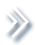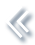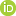####ArchivesReview Articles

## On studying extreme values and systematic risks with nonlinear time series models and tail dependence measures

Zhengjun Zhangzjz@stat.wisc.edu

Pages 1-25 | Received 10 Apr. 2020, Accepted 24 Nov. 2020, Published online: 23 Dec. 2020,
• Abstract
• References
• Citations

## ABSTRACT

This review paper discusses advances of statistical inference in modeling extreme observations from multiple sources and heterogeneous populations. The paper starts briefly reviewing classical univariate/multivariate extreme value theory, tail equivalence, and tail (in)dependence. New extreme value theory for heterogeneous populations is then introduced. Time series models for maxima and extreme observations are the focus of the review. These models naturally form a new system with similar structures. They can be used as alternatives to the widely used ARMA models and GARCH models. Applications of these time series models can be in many fields. The paper discusses two important applications: systematic risks and extreme co-movements/large scale contagions.

## References

1. Beirlant, J., Goegebeur, Y., Segers, J., & Teugels, J. (2006). Statistics of extremes: Theory and applications. John Wiley & Sons. [Google Scholar]
2. Brown, B. M., & Resnick, S. I. (1977). Extreme values of independent stochastic processes. Journal of Applied Probability14(4), 732–739. https://doi.org/10.2307/3213346 [Crossref][Web of Science ®], [Google Scholar]
3. Bücher, A., & Segers, J. (2017). On the maximum likelihood estimator for the generalized extreme-value distribution. Extremes20(4), 839–872. https://doi.org/10.1007/s10687-017-0292-6 [Crossref][Web of Science ®], [Google Scholar]
4. Cao, W., & Zhang, Z. (2020). New extreme value theory for maxima of maxima. Statistical Theory and Related Fieldshttps://doi.org/10.1080/24754269.2020.1846115 [Taylor & Francis Online], [Google Scholar]
5. Cartwright, D. E. (1958). On estimating the mean energy of sea waves from the highest waves in a record. Proceedings of the Royal Society of London, Series A247(1248), 22–28.https://doi.org/10.1098/rspa.1958.0168 [Google Scholar]
6. Castillo, E., Hadi, A. S., Balakrishnan, N., & Sarabia, J. M. (2005). Extreme value and related models with applications in engineering and science. Wiley Series in Probability and Statistics. [Google Scholar]
7. Chen, Y., Wang, Z. C., & Zhang, Z. (2019). Mark to market value at risk. Journal of Econometrics208(1), 299–321. https://doi.org/10.1016/j.jeconom.2018.09.017 [Crossref][Web of Science ®], [Google Scholar]
8. Coles, S., Bawa, J., Trenner, L., & Dorazio, P. (2001). An introduction to statistical modeling of extreme values (vol. 208). Springer. [Crossref], [Google Scholar]
9. Coles, S., Heffernan, J., & Tawn, J. (1999). Dependence measures for extreme value analyses. Extremes2(4), 339–365. https://doi.org/10.1023/A:1009963131610 [Crossref], [Google Scholar]
10. Coles, S. G., & Tawn, J. A. (1991). Modeling extreme multivariate events. Journal of the Royal Statistical Society, Series B53(2), 377–392.https://doi.org/10.1111/j.2517-6161.1991.tb01830.x [Google Scholar]
11. Davis, R. A., & Resnick, S. I. (1989). Basic properties and prediction of max-ARMA processes. Advances in Applied Probability21(4), 781–803. https://doi.org/10.2307/1427767 [Crossref][Web of Science ®], [Google Scholar]
12. Davis, R. A., & Resnick, S. I. (1993). Prediction of stationary max-stable processes. The Annals of Applied Probability3(2), 497–525. https://doi.org/10.1214/aoap/1177005435 [Crossref], [Google Scholar]
13. de Haan, L. (1984). A spectral representation for max-stable processes. The Annals of Probability12(4), 1194–1204. https://doi.org/10.1214/aop/1176993148 [Crossref][Web of Science ®], [Google Scholar]
14. de Haan, L. (1985). Extremes in higher dimensions: The model and some statistics. In Proceedings of the 45th session international statistic institute. International Statistical Institute. [Google Scholar]
15. de Haan, L. (1993). Extreme value statistics. In Janos Galambos, James Lechner, & Emil Simiu (Eds.), Extreme value theory and applications (pp. 93–122). Kluwer Academic. [Google Scholar]
16. de Haan, L., & Ferreira, A. (2007). Extreme value theory: An introduction. Springer. [Google Scholar]
17. de Haan, L., & Resnick, S. I. (1977). Limit theory for multivariate sample extremes. Zeitschrift für Wahrscheinlichkeitstheorie und Vrwandte Gebiete40(4), 317–337. https://doi.org/10.1007/BF00533086 [Crossref], [Google Scholar]
18. Deheuvels, P. (1983). Point processes and multivariate extreme values. Journal of Multivariate Analysis13(2), 257–272. https://doi.org/10.1016/0047-259X(83)90025-8 [Crossref][Web of Science ®], [Google Scholar]
19. Deng, L., & Zhang, Z. (2018). Assessing the features of extreme smog in China and the differentiated treatment strategy. Proceedings of the Royal Society A474, 220920170511. https://doi.org/10.1098/rspa.2017.0511 [Crossref], [Google Scholar]
20. Deng, L., & Zhang, Z. (2020). The haze extreme co-movements in Beijing-Tianjin-Hebei region and its extreme dependence pattern recognitions. Science Progress103(2), 36850420916315. https://doi.org/10.1177/0036850420916315 [Crossref][Web of Science ®], [Google Scholar]
21. Dey, D. K., & Yan, J. (2016). Extreme value modeling and risk analysis: Methods and applications, (EDS). Chapman & Hall/CRC. [Crossref], [Google Scholar]
22. Drees, H., Ferreira, A., & de Haan, L. (2004). On maximum likelihood estimation of the extreme value index. Annals of Applied Probability14(3), 1179–1201. https://doi.org/10.1214/105051604000000279 [Crossref][Web of Science ®], [Google Scholar]
23. Embrechts, P., Klüppelberg, C., & Mikosch, T. (1997). Modelling extremal events for insurance and finance. Springer. [Crossref], [Google Scholar]
24. Embrechts, P., Lindskog, F., & McNeil, A. (2003). Modelling dependence with copulas and applications to risk management. In S. Rachev (Ed.), Handbook of heavy tailed distributions in finance (pp. 329–384). Elsevier. [Crossref], [Google Scholar]
25. Embrechts, P., McNeil, A., & Straumann, D. (2002). Correlation and dependence in risk management: Properties and pitfalls. In M. A. H. Dempster (Ed.), Risk management: Value at risk and beyond (pp. 176–223). Cambridge University Press. [Google Scholar]
26. Embrechts, P., Resnick, S. I., & Samorodnitsky, G. (1999). Extreme value theory as a risk management tool. North American Actuarial Journal3(2), 30–41. https://doi.org/10.1080/10920277.1999.10595797 [Taylor & Francis Online], [Google Scholar]
27. Ferreira, H., & Ferreira, M. (2018). Estimating the extremal index through local dependence. Annales de l'Institut Henri Poincaré, Probabilités et Statistiques54(2), 587–605. https://doi.org/10.1214/16-AIHP815 [Crossref][Web of Science ®], [Google Scholar]
28. Finkenstädt, B., & Rootzén, H. E. (2004). Extreme values in finance, telecommunications, and the environment. Chapman & Hall/CRC. [Google Scholar]
29. Fougéres, A.-L., Nolan, J. P., & Rootzén, H. (2009). Models for dependent extremes using stable mixtures. Scandinavian Journal of Statistics36, 42–59. https://doi.org/10.1111/j.1467-9469.2008.00613.x [Web of Science ®], [Google Scholar]
30. Galambos, J. (1987). Asymptotic theory of extreme order statistics (2nd ed.). Krieger. [Google Scholar]
31. Hall, P., Peng, L., & Yao, Q. (2002). Moving-maximum models for extrema of time series. Journal of Statistical Planning and Inference103(1–2), 51–63. https://doi.org/10.1016/S0378-3758(01)00197-5 [Crossref][Web of Science ®], [Google Scholar]
32. Heffernan, J. E. (2001). A directory of coefficients of tail dependence. Extremes3(3), 279–290. https://doi.org/10.1023/A:1011459127975 [Crossref], [Google Scholar]
33. Heffernan, J. E., Tawn, J. A., & Zhang, Z. (2007). Asymptotically (in)dependent multivariate maxima of moving maxima processes. Extremes10(1-2), 57–82. https://doi.org/10.1007/s10687-007-0035-1 [Crossref], [Google Scholar]
34. Hsing, T. (1993). Extremal index estimation for a weakly dependent stationary sequence. The Annals of Statistics21(4), 2043–2071. https://doi.org/10.1214/aos/1176349409 [Crossref][Web of Science ®], [Google Scholar]
35. Huser, R., & Davison, A. C. (2013). Composite likelihood estimation for the Brown–Resnick process. Biometrika100(2), 511–518. https://doi.org/10.1093/biomet/ass089 [Crossref][Web of Science ®], [Google Scholar]
36. Hüsler, J., & Li, D. (2009). Testing asymptotic independence in bivariate extremes. Journal of Statistical Planning and Inference139(3), 990–998. https://doi.org/10.1016/j.jspi.2008.06.003 [Crossref][Web of Science ®], [Google Scholar]
37. Idowu, T., & Zhang, Z. (2017). An extended sparse max-linear moving model with application to high-frequency financial data. Statistical Theory and Related Fields1(1), 92–111. https://doi.org/10.1080/24754269.2017.1346852 [Taylor & Francis Online], [Google Scholar]
38. Joe, H. (2014). Dependence modeling with copulas. Chapman & Hall/CRC Monographs on Statistics & Applied Probability. Chapman & Hall/CRC. [Crossref], [Google Scholar]
39. Kelly, B. (2014). The dynamic power law model. Extremes17(4), 557–583. https://doi.org/10.1007/s10687-014-0193-x [Crossref][Web of Science ®], [Google Scholar]
40. Kunihama, T., Omori, Y., & Zhang, Z. (2012). Efficient estimation and particle filter for max-stable processes. Journal of Time Series Analysis33(1), 61–80. https://doi.org/10.1111/jtsa.2011.33.issue-1 [Crossref][Web of Science ®], [Google Scholar]
41. Leadbetter, M. R. (1983). Extremes and local dependence in stationary sequences. Zeitschrift für Wahrscheinlichkeitstheorie und Verwandte Gebiete65(2), 291–306. https://doi.org/10.1007/BF00532484 [Crossref][Web of Science ®], [Google Scholar]
42. Leadbetter, M. R., Lindgren, G., & Rootzén, H. (1983). Extremes and related properties of random sequences and processes. Springer Science & Business Media. [Crossref], [Google Scholar]
43. Leadbetter, M. R., Weissman, I., de Haan, L., & Rootzén, H. (1989). On clustering of high values in statistically stationary seriess. In J. Sanson (Ed.), Proceedings of the 4th international meeting on statistical climatology. New Zealand Meteorological Service. [Google Scholar]
44. Ledford, A. W., & Tawn, J. A. (1996). Statistics for near independence in multivariate extreme values. Biometrika83(1), 169–187. https://doi.org/10.1093/biomet/83.1.169 [Crossref][Web of Science ®], [Google Scholar]
45. Ledford, A. W., & Tawn, J. A. (1997). Modeling dependence within joint tail regions. Journal of the Royal Statistical Society: Series B (Statistical Methodology)59(2), 475–499. https://doi.org/10.1111/rssb.1997.59.issue-2 [Crossref], [Google Scholar]
46. Loynes, R. M. (1965). Extreme values in uniformly mixing stationary stochastic processes. The Annals of Mathematical Statistics36(3), 993–999. https://doi.org/10.1214/aoms/1177700071 [Crossref], [Google Scholar]
47. Mao, G., & Zhang, Z. (2018). Stochastic tail index model for high frequency financial data with Bayesian analysis. Journal of Econometrics205(2), 470–487. https://doi.org/10.1016/j.jeconom.2018.03.019 [Crossref][Web of Science ®], [Google Scholar]
48. Martins, A. P., & Ferreira, H. (2014). Extremal properties of M4 processes. Test23(2), 388–408. https://doi.org/10.1007/s11749-014-0358-6 [Crossref][Web of Science ®], [Google Scholar]
49. Massacci, D. (2016). Tail risk dynamics in stock returns: Links to the macroeconomy and global markets connectedness. Management Science63(9), 1–18.https://doi.org/10.1287/mnsc.2016.2488 [Web of Science ®], [Google Scholar]
50. McNeil, A. J., & Frey, R. (2000). Estimation of tail-related risk measures for heteroscedastic financial time series: An extreme value approach. Journal of Empirical Finance7(3–4), 271–300. https://doi.org/10.1016/S0927-5398(00)00012-8 [Crossref], [Google Scholar]
51. Meinguet, T. (2012). Maxima of moving maxima of continuous functions. Extremes15(3), 267–297. https://doi.org/10.1007/s10687-011-0136-8 [Crossref][Web of Science ®], [Google Scholar]
52. Mikosch, T., Embrechts, P., & Klüppelberg, C. (1997). Modelling extremal events for insurance and finance. Springer Verlag. [Google Scholar]
53. Nandagopalan, S. (1990). Multivariate extremes and the estimation of the extremal index [PhD thesis]. University of North Carolina, Chapel Hill, Dept. Of Statistics. [Google Scholar]
54. Nandagopalan, S. (1994). On the multivariate extremal index. Journal of Research of the National Institute of Standards and Technology99(4), 543–550. https://doi.org/10.6028/jres.099.052 [Crossref][Web of Science ®], [Google Scholar]
55. Naveau, P., Zhang, Z., & Zhu, B. (2011). An extension of max autoregressive models. Statistics and Its Interface4(2), 253–266. https://doi.org/10.4310/SII.2011.v4.n2.a19 [Crossref][Web of Science ®], [Google Scholar]
56. Newell, G. F. (1964). Asymptotic extremes for m-dependent random variables. The Annals of Mathematical Statistics35(3), 1322–1325. https://doi.org/10.1214/aoms/1177703288 [Crossref], [Google Scholar]
57. O'Brien, G. L. (1974). Limit theorems for the maximum term of a stationary process. Annals of Probability2(3), 540–545. https://doi.org/10.1214/aop/1176996673 [Crossref][Web of Science ®], [Google Scholar]
58. O'Brien, G. L. (1987). Extreme values for stationary and Markov sequences. Annals of Probability15(1), 281–291. https://doi.org/10.1214/aop/1176992270 [Crossref][Web of Science ®], [Google Scholar]
59. Peng, L. (1999). Estimation of the coefficient of tail dependence in bivariate extremes. Statistics & Probability Letters43(4), 399–409. https://doi.org/10.1016/S0167-7152(98)00280-6 [Crossref][Web of Science ®], [Google Scholar]
60. Pereira, L., & Fonseca, C. (2019). Statistical methods for assessing the contagion of spatial extreme events among regions. Communications in Statistics - Theory and Methods48(13), 3208–3218. https://doi.org/10.1080/03610926.2018.1473612 [Taylor & Francis Online][Web of Science ®], [Google Scholar]
61. Pickands, J. (1981). Multivariate extreme value distributions. In Proceedings 43rd session of the international statistical institute (Vol. 49, pp. 859–878). [Google Scholar]
62. Reich, B. J., & Shaby, B. A. (2019). A spatial Markov model for climate extremes. Journal of Computational and Graphical Statistics28(1), 117–126. https://doi.org/10.1080/10618600.2018.1482764 [Taylor & Francis Online][Web of Science ®], [Google Scholar]
63. Resnick, S. I. (1987). Extreme values, regular variation, and point processes. Springer. [Crossref], [Google Scholar]
64. Rodgers, J. L., & Nicewander, W. A. (1988). Thirteen ways to look at the correlation coefficient. American Statistician42(1), 59–66. https://doi.org/10.2307/2685263 [Crossref][Web of Science ®], [Google Scholar]
65. Salmon, F. (2012). The formula that killed wall street. Significance9(1), 16–20. https://doi.org/10.1111/j.1740-9713.2012.00538.x [Crossref], [Google Scholar]
66. Salvadori, G., De Michele, C., Kottegoda, N. T., & Rosso, R. (2007). Extremes in nature: An approach using copulas. Springer. Complexity. [Crossref], [Google Scholar]
67. Sibuya, M. (1959). Bivariate extreme statistics, I. Annals of the Institute of Statistical Mathematics11(2), 195–210. [Google Scholar]
68. Smith, R. L. (1985). Maximum likelihood estimation in a class of nonregular cases. Biometrika72(1), 67–90. https://doi.org/10.1093/biomet/72.1.67 [Crossref][Web of Science ®], [Google Scholar]
69. Smith, R. L. (1990). Extreme value theory. Handbook of Applicable Mathematics7, 437–471. [Google Scholar]
70. Smith, R. L., & Weissman, I. (1994). Estimating the extremal index. Journal of the Royal Statistical Society, Series B56(3), 515–528. [Google Scholar]
71. Smith, R. L., & Weissman, I. (1996). Characterization and estimation of the multivariate extremal index. Technical report, University of North Carolina-Chapel Hill. [Google Scholar]
72. Tang, R., Shao, J., & Zhang, Z. (2013). Sparse moving maxima models for tail dependence in multivariate financial time series. Journal of Statistical Planning and Inference143(5), 882–895. https://doi.org/10.1016/j.jspi.2012.11.008 [Crossref][Web of Science ®], [Google Scholar]
73. Tawn, J. A. (1988). Bivariate extreme value theory: Models and estimation. Biometrika75(3), 397–415. [Crossref][Web of Science ®], [Google Scholar]
74. Weissman, I. (1994). On the extremal index of stationary sequences. Technical report, The Conference on Multivariate Extreme Value Estimation with Applications to Economics and Finance, Erasmus University, Rotterdam. [Google Scholar]
75. Wu, J., Zhang, Z., & Zhao, Y. (2012). Study of the tail dependence structure in global financial markets using extreme value theory. Journal of Reviews on Global Economics1(1), 62–81. [Google Scholar]
76. Yang, X., Frees, E. W., & Zhang, Z. (2011). A generalized beta copula with applications in modeling multivariate long-tailed data. Insurance: Mathematics and Economics49(2), 265–284. https://doi.org/10.1016/j.insmatheco.2011.04.007 [Crossref][Web of Science ®], [Google Scholar]
77. Zhang, Z. (2005). A new class of tail-dependent time series models and its applications in financial time series. Advances in Econometrics20(B), 323–358. [Google Scholar]
78. Zhang, Z. (2008a). The estimation of M4 processes with geometric moving patterns. Annals of the Institute of Statistical Mathematics60(1), 121–150. https://doi.org/10.1007/s10463-006-0078-0 [Crossref][Web of Science ®], [Google Scholar]
79. Zhang, Z. (2008b). Quotient correlation: A sample based alternative to Pearson's correlation. The Annals of Statistics36(2), 1007–1030. https://doi.org/10.1214/009053607000000866 [Crossref][Web of Science ®], [Google Scholar]
80. Zhang, Z. (2009). On approximating max-stable processes and constructing extremal copula functions. Statistical Inference for Stochastic Processes12(1), 89–114. https://doi.org/10.1007/s11203-008-9027-2 [Crossref], [Google Scholar]
81. Zhang, Z., Qi, Y., & Ma, X. (2011). Asymptotic independence of correlation coefficients with application to testing hypothesis of independence. Electronic Journal of Statistics5, 342–372. https://doi.org/10.1214/11-EJS610 [Crossref][Web of Science ®], [Google Scholar]
82. Zhang, X., & Schwaab, B. (2017). Tail risk in government bond markets and ECB asset purchases. Working paper. [Google Scholar]
83. Zhang, Z., & Smith, R. L. (2004). The behavior of multivariate maxima of moving maxima processes. Journal of Applied Probability41(4), 1113–1123. https://doi.org/10.1239/jap/1101840556 [Crossref][Web of Science ®], [Google Scholar]
84. Zhang, Z., & Smith, R. L. (2010). On the estimation and application of max-stable processes. Journal of Statistical Planning and Inference140(5), 1135–1153. https://doi.org/10.1016/j.jspi.2009.10.014 [Crossref][Web of Science ®], [Google Scholar]
85. Zhang, Z., Zhang, C., & Cui, Q. (2017). Random threshold driven tail dependence measures with application to precipitation data analysis. Statistica Sinica27(2), 685–709.https://doi.org/10.5705/ss.202015.0421 [Web of Science ®], [Google Scholar]
86. Zhang, Z., & Zhu, B. (2016). Copula structured M4 processes with application to high-frequency financial data. Journal of Econometrics194(2), 231–241. https://doi.org/10.1016/j.jeconom.2016.05.004 [Crossref][Web of Science ®], [Google Scholar]
87. Zhao, Z., & Zhang, Z. (2018). Semi-parametric dynamic max-copula model for multivariate time series. Journal of the Royal Statistical Society: Series B (Statistical Methodology)80(2), 409–432. https://doi.org/10.1111/rssb.12256 [Crossref][Web of Science ®], [Google Scholar]
88. Zhao, Z., Zhang, Z., & Chen, R. (2018). Modeling maxima with autoregressive conditional Fréchet model. Journal of Econometrics207, 2325–351. https://doi.org/10.1016/j.jeconom.2018.07.004 [Crossref][Web of Science ®], [Google Scholar]
89. Zhou, C. (2008). A two-step estimator of the extreme value index. Extremes11(3), 281–302. https://doi.org/10.1007/s10687-008-0058-2 [Crossref][Web of Science ®], [Google Scholar]

Jingyu Ji, Deyuan Li. (2021) Application of autoregressive tail-index model to China's stock marketStatistical Theory and Related Fields 5:1, pages 31-34.

Zhengjun Zhang. (2021) Rejoinder of “On studying extreme values and systematic risks with nonlinear time series models and tail dependence measures”Statistical Theory and Related Fields 5:1, pages 45-48.

## Articles from other publishers

Guanqi Fang, Rong Pan. (2021) On multivariate copula modeling of dependent degradation processes. Computers & Industrial Engineering 159, pages 107450.
Crossref

Zhengjun Zhang. (2021) Five Critical Genes Related to Seven COVID-19 Subtypes: A Data Science Discovery. Journal of Data Science 26, pages 142-150.
Crossref